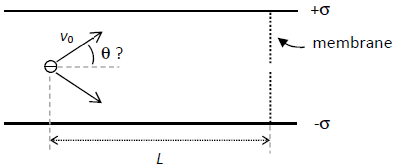# Electron between two parallel plates

Mike94
Homework Statement:
An electron is thrown halfway between two large plates, with an angle Theta and an initial speed v0. The resultant electric field caused by the plates is vertical and its magnitude is noted by E. A neutral and insulating vertical membrane located to the right of the electron has an opening halfway between the two plates.
V0 = 5 * 10^6 m/s
E = 550 N/C
L = 25 cm
Relevant Equations:
a) Calculate the value of the parameter s ( sigma )
b) Say if the electron should be projected at an up angle (theta> 0) or down (theta <0) to pass through the opening in the membrane. Justify your answer.
c) Consequently to your answer in b), calculate the two possible angle values in order to that the electron can exit through the opening.a) E = s / E0 so s is 4.87E-9
b) The electron will be projected at up angle since its charge is negative ( not sure if there's another reason behind it)

c)

Initial speed:

V0 = 5 * 10^6 * cos(theta) + 5 * 10^6 * sin(theta)

The force suffered by the electron is:

Fy = q*Ey

Fy = -1.602*10^19 * -550 N/C

Fy = 8.811E-17

The acceleration of the electron:

Fy = m*ay

8.811E-17 = 9.11*10^-31 * ay

Ay = 9.6718E13

The time it takes for the electron to exit

X= x0 + v0x * t

0.25 = 0 m + 5 * 10^6 * t

t = 5 * 10-E8

The speed that the electron quits the plates

Vy = v0y + ay * t

Vy = 0 + 9.6718E13 * 5 * 10-E8

Vy = 4835894.62

I’m not sure if I’m on the right path or not.

I think the way I need to find the angle is like:

Theta = artcan ( vy / vx )

Thank you !

Homework Helper
Gold Member
2022 Award
a) E = s / E0 so s is 4.87E-9
...
Fy = 8.811E-17
...
Ay = 9.6718E13
Units!
b) The electron will be projected at up angle since its charge is negative ( not sure if there's another reason behind it)
That's not what the question asked.
c)Initial speed:
V0 = 5 * 10^6 * cos(theta) + 5 * 10^6 * sin(theta)
Without vectors in the equation it makes no sense.
The time it takes for the electron to exit
X= x0 + v0x * t
0.25 = 0 m + 5 * 10^6 * t
v0=5 * 10^6m/s. What is v0x?
The speed that the electron quits the plates
Can you see a symmetry here?
I think the way I need to find the angle is like:
Theta = artcan ( vy / vx )
Yes, or use the symmetry l

Mike94
Units!

E = 505 N/C
E0 = 8.854 * 10^-12 C^2 / (Nm^2)

E = s / E0
So my s should be 4.87E-9 uC / m^2

Fy = qE
Fy = -1.602 * 10E-19 * 550 N/C
Fy = 8.881E-17 N

Fy = may
8.811E-17 N = 9.11 * 10E-31 kg * ay
ay = 9.6718E13 m/s^2

x = x0 + v0x * t
0.25 m = 0 m + 5*10^6 * t
t = 5E-8 s

vy = v0y + ay * t
vy = 0 + 9.6718E13 * 5E-8
vy = 4835894.6213 m/2

Theta = arctan(4835894.6213/5E6)
Theta = 44 degrees

Can you see a symmetry here?

So I guess you mean what I get on the exit, that's my theta at the beginning ?

The other way I see it:

Initial speed:
V0 = 5 * 10^6 * cos(theta) i + 5 * 10^6 * sin(theta) j

x = x0 + vx0t + 1/2axt^2 => t = x-x0 / vx0 => 0.25 m - 0 m/ 5E6*cos(theta)
vy = vy0 + ayt => vy = 5E6*sin(theta) + 9.6718E13 m/s^2 * x
theta = arctan(vy / 5E6*cos(theta))

Mike94
After working for on it, I see it as a projectile:

R=v0^2*sin(2*theta) / |ay| => 0.25 m = 5E6^2 * sin(2*theta) / 9.6718E13 m/s^2
theta equals to 37.64 degrees or 52.3599 degrees

Last edited:
Homework Helper
Gold Member
2022 Award
E = 505 N/C
E0 = 8.854 * 10^-12 C^2 / (Nm^2)

E = s / E0
So my s should be 4.87E-9 uC / m^2
That's an extremely small charge. Check exponent/units.
x = x0 + v0x * t
0.25 m = 0 m + 5*10^6 * t
You seem to have missed my comment:
v0=5 * 10^6m/s. What is v0x?
But you have sidestepped that error by using:
After working for on it, I see it as a projectile:

R=v0^2*sin(2*theta) / |ay| => 0.25 m = 5E6^2 * sin(2*theta) / 9.6718E13 m/s^2
theta equals to 37.64 degrees or 52.3599 degrees
Quite so... as a projectile it 'lands' at its launch height, so vertical velocity is just reversed.

Mike94
That's an extremely small charge. Check exponent/units.

I meant to say that "s" is equals to 4.8697E-9 C

Quite so... as a projectile it 'lands' at its launch height, so vertical velocity is just reversed.

Not quite sure what you meant by vertical velocity is just reversed. Do you mean that my final angle will be 90 degrees minus the angles from the final vertical component.

Thanks.

•